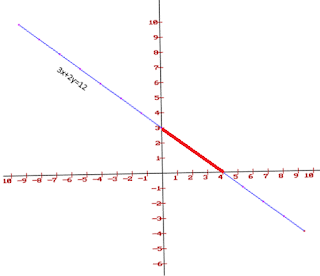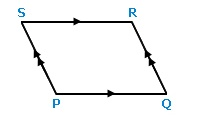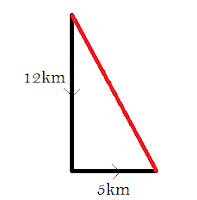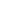2015 SSC Solved Quantitative Aptitude Questions- WIth Detailed explanation

1. If the length of the portion of the straight line 3x+4y =12  intercepted between the axes is:
a) 3
b) 4
c) 7
d) 5

Ans:dSolution
To solve x-intercept, Let y=0.
3x+0 = 12
x=4

To solve y-intercept,let x=0.

0+4y=12
y=3

The line passes through (4,0) in x axis , and  (0,3) in y axis.

Use equation:x2=4, x1=0 ,y2 =3,y1=0
Ans: 5

2.What is the sum of first 'n' natural numbers?

Ans: n(n+1)/2

3. The perimeter of one face of a cube is 20 cm. Its volume will be :

a) 100 cm3
b) 125 cm3
c) 400 cm3
d) 625 cm3
Ans:b

Solution:

Let side of cube = a

Perimeter of one face = 4a =20cm
So , a=5 cm
Volume = a3 = 53 =125 cm3

4. If 10% discount and then 20 % discount in succession is equiavalent to total of:

a) 15%
b) 30%
c) 24%
d) 28%
Ans: d
Solution:
If the first discount is x% and 2nd discount is y% then ,

Total discount = ( x + y - xy / 100 ) %

x = 10% and y = 20%
Total discount = [ 10 + 20 – ( 10 x 20) / 100] %
= ( 30 – 200 /100 ) %

Total discount = 28%

5. Allowing 20% and 15% successive discounts,the selling price of an article becomes ₹3060; then the marked price will be:

a) ₹4400   b) ₹5000
c) ₹4500   d) ₹4000
Ans: c

Solution:

Total discount = [ 20 + 15 – ( 20 x 15) / 100] % .

= ( 35 – 3 ) % = 32%
Selling price = Marked Price - Discount

Rs 3060 = MP - MP(32/100)

3060 = MP(68/100
MP= Rs. 4500

6. Eighteen years ago,the ratio of A's age to B's age was 8:13. Their Present ratio's are 5:7. What is the present age of A?

a) 70 years  b) 50 years
c) 40 years  d) 60 years
Ans: b

Solution:

Eight years ago, let age of A = 8x
Then age of B will be 13x
After 8 years(present);  (8x+18)/(13x+18) = 5/7
7(8x+18) = 5/(13x+18)
56x+126 = 65x+90
36x = 9 ; x=4
Present age of A = (8x+18) =50

7. If m= -4 ,n =-2, then the value of m- 3m2 + 3m + 3n + 3n2 + n3 is

Ans: -126 (Direct substitution)

8. In a parellogram PQRS, angle P is four times of angle Q,then the measure of R ?

a) 36 degree     b) 72 degree

c) 130 degree   d) 144 degree
Ans: d
Solution:For a parallelogram , consecutive angles are supplementary (P + Q = 180°).

P +∠Q = 180°
4∠Q + ∠Q =180° (Since angle P is four times angle Q)
5(∠Q) = 180 degree
∠Q = 180/5 =36 degree
Therefore ∠P =144 degree
For paralleogram opposite angles are equal. So ∠R =144 degree

9. The population of a town increases by 5% every year .If the present population is 9261, the population 3 years ago was:

Ans: 8000
Solution:
This can be solved by Compound interest equation.
CI= 9261
R=5%
n=3
CI= P(1+ R/100)n

10. The average weight of 15 oarsmen in a boat is increased by 1.6kg when one of the crew,who weighs 42kg is replaced by a new man. Find the weight of new man.(in kg).
Ans: 66 kg.
Solution:
It is clear that new man has more weight than earlier one.
Total increase in weight= 1.6kg x 15 = 24kg
Weight of new man = 42+24= 66kg.

11. the sides of a triangle having area 7776 sq.cm are in the ratio 3:4:5. the perimeter of the traingle is :
Ans: 432cm.
Solution:
Let the length of sides be 3x , 4x, 5x
Side 5x is the hypotenuse.
Area = 1/2bh
= 1/2 (3x)(4x) = 6x2

So, 6x2 =7776
Solving x=36.
Length of Sides are 108cm, 144cm , 180cm.
Perimeter = 108+144+180 = 432sm.

12. The measure of an angle whose supplement is three times as large as its complement, is
a)30 degree     b) 45 degree
c) 60 degree    d) 75 degree
Ans: 45 degree
12. FInd the value of tan4tan43tan47tan86
Ans: 1
Solution
tan(86) = tan(90-86) =cot(4)
tan(47) = tan(90-47) = cot (43)
so, tan4 tan 43 cot 43 cot 4 =  (tan4 cot 4 )( tan 43 cot 43) =1

13. A ship after sailing 12 km towards south from a particular place covered 5km more towards east. Then the straight way distance of the ship from the place is :
Ans: 13km

Solution:The distance will be the length of line joining initial points.

It can be found out by pythagoras theorem.

Distance = √(122 + 52)

= 13.

14. Two poles of height 7m and 12m stand on plane ground. If the distance between the feet is 12m, the distance between their top will be:

Ans: 13m

Solution:

AB=12m
BC = 12-7 =5m
AC= Distance between tops
Distance between tops,AC = √(122 + 52)

= 13m

15.  The marked price of a watch was Rs.720. A man bought the same for Rs 550.80 after getting two discounts, the first being 10%. The second discount rate is:

Ans: 15%

Solution:

Total Discount = (720-550.8)/720 = .235
= 23.5 %

Total discount = ( x + y - xy / 100 ) %
23.5 =  ( 10 + y - 10y / 100 )
9y/10 = 13.5
y=15

16. If x+1/x =2.then the value of x12 - 1/ x12 is :
Ans: 0

Solution:
x+1/x =2
x2 - 2x + 1 =0
x=1

COMING SOON- Remaining Questions will be solved later.Visit tomorrow
Share :
+
Next
« Prev
Prev
Next »

Featured

Modi Government Schemes and Programs-GK Questions and Answers